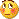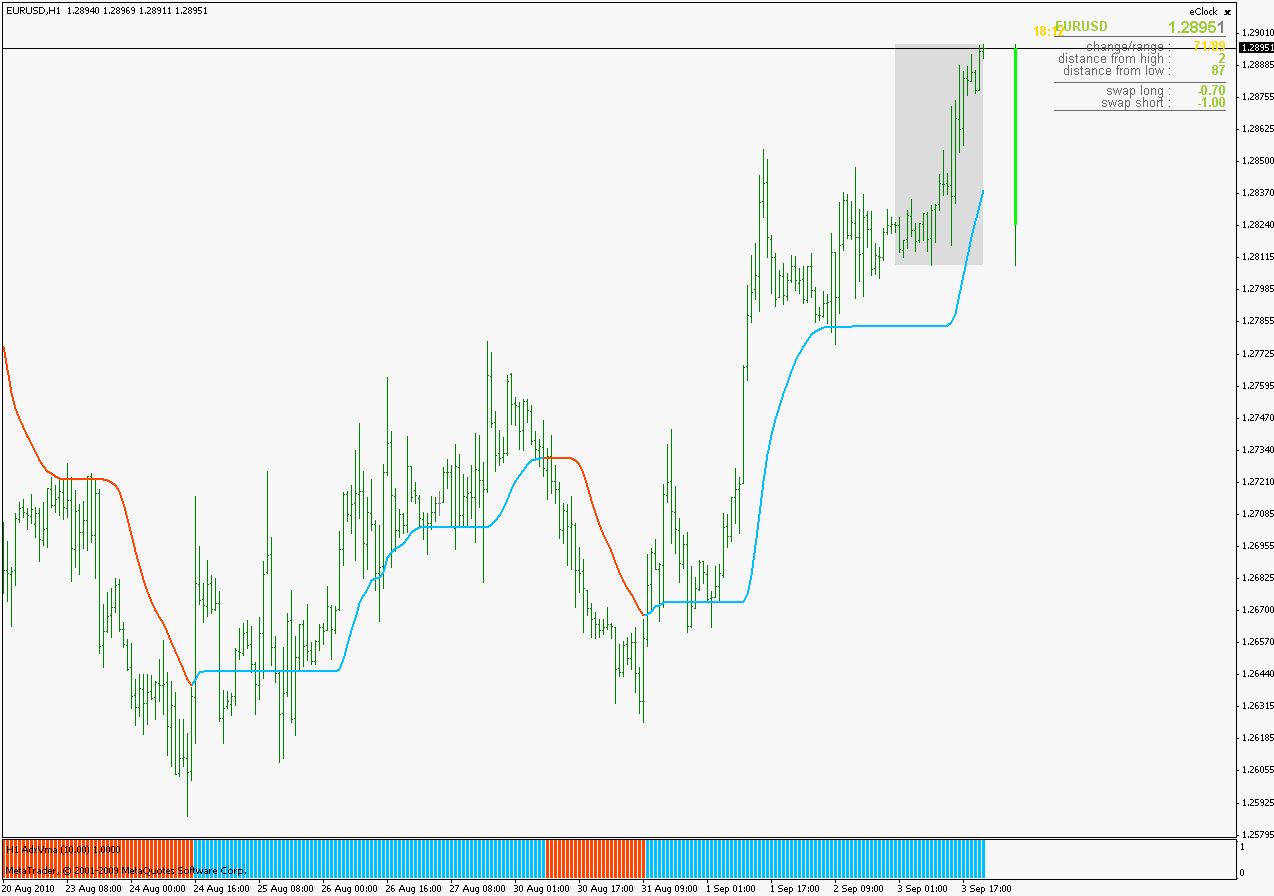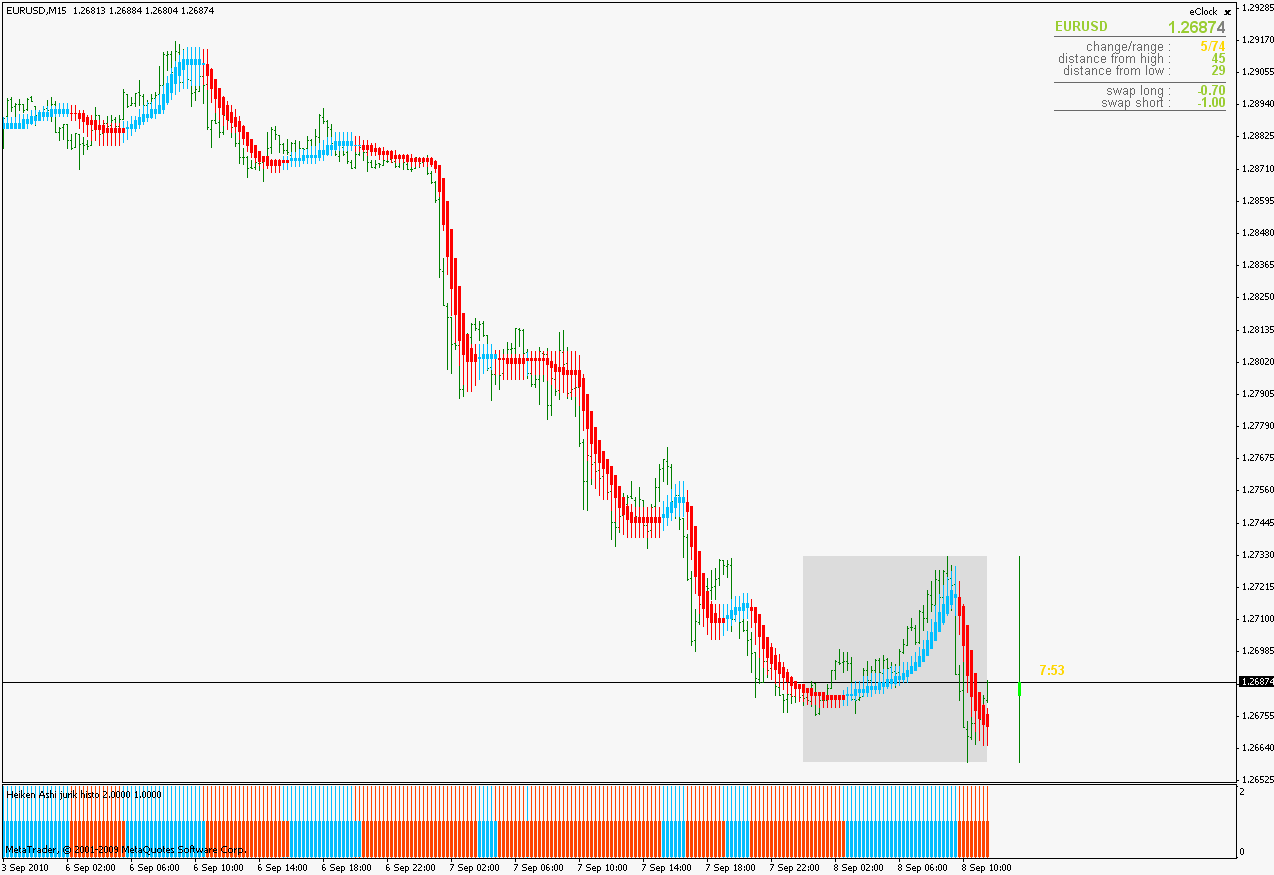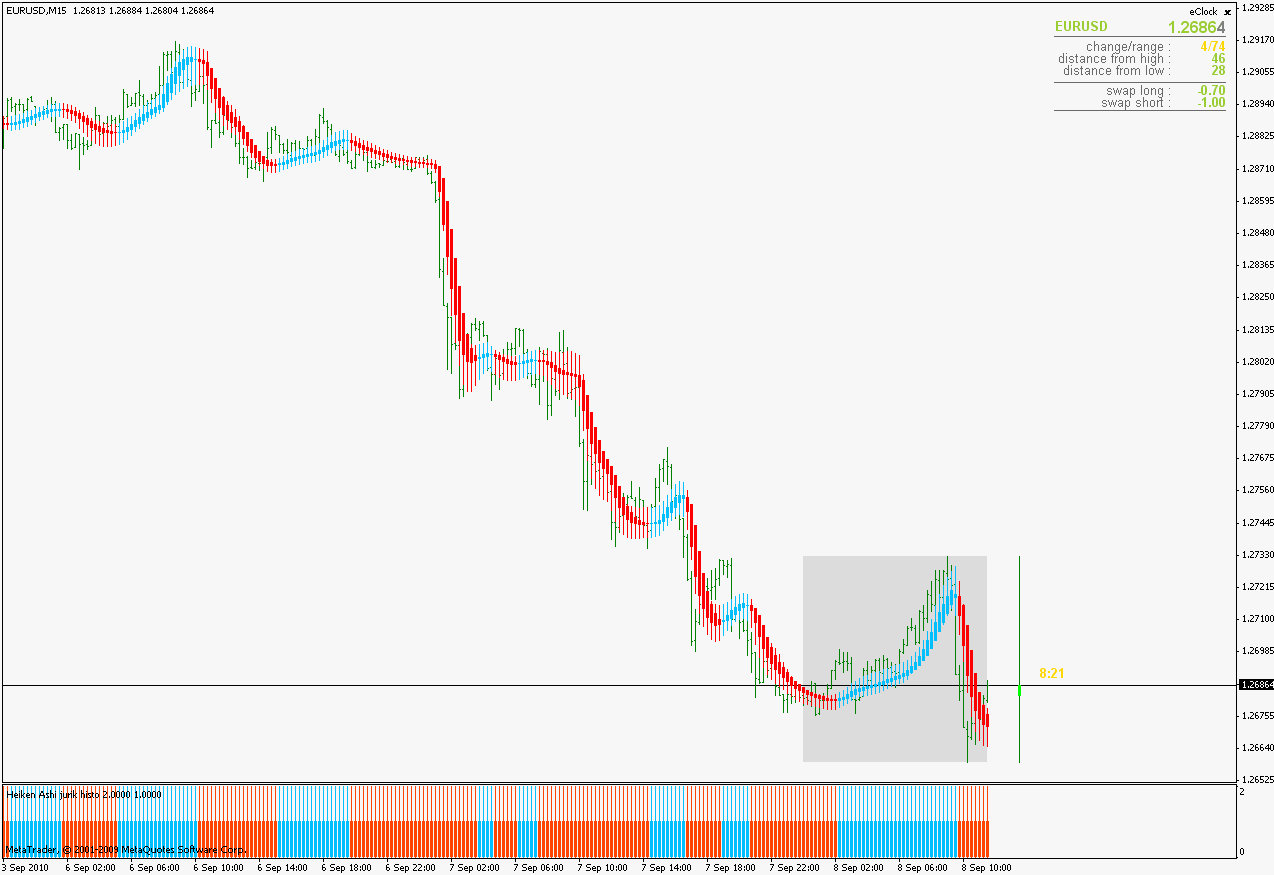Elite indicators :) - page 185531

Would I be out of line to ask you to post the Tradestation version??

Ray

Guys, I have noticed one illogical thing in the indicator (the first step how adx part is calculated) I did use tradestation indicator as a model and it seems that there is an error in it that I inherited without thinking of it. In these that error is corrected. Even the results are better this way.This calculation is much closer to the one from the public section (so the one from the public section is a fairly correct indicator) with much faster code in 99% of time and extras that are specific just to these posted in the elite section. So if you downloaded the indicators from previous posts, please use these instead. Also added one more option to the "regular" version : MultiColorMode- if set to false just one color is going to be used to display adxvma (useful if one wants to use a couple of adxvma to check for crosses as signals)

regards151619

Ray,

Here it is. The indicator :

inputs:

Price (close),

length (14);

vars:

av (0);

av = ADXVMA(Price, length);

Plot1(av, "Rising");

Plot2(av, "Falling");

Plot3(av, "Neutral");

Plot4(av, "ADXVMA", iff(av = av, GetPlotColor(3), iff(av > av, GetPlotColor(1), GetPlotColor(2))));

NoPlot(1);

NoPlot(2);

NoPlot(3); [/php]and the adxvma function

[php]inputs:

Price (NumericSeries),

Length (NumericSimple);

vars:

TR(0),

DI_Diff(0),

DI_Sum(0),

ma(0),

pdm(0),

mdm(0),

pdi(0),

mdi(0),

DI_Factor(0),

VI(0),

diff(0),

HHV(0),

LLV(0),

WeightDM(Length),

WeightDI(Length),

WeightDX(Length),

ChandeEMA(Length),

out(0),

j(0);

once ma=Price;

//

// in order to make it right the "pdm=0; mdm=0;" must be added (it does not

// exist in original and that is an error, tradestation inherits values fom

// a previous loop and they must be zeroed before these calculations)

//

pdm=0; mdm=0;

if(Price>Price) then pdm=Price-Price else mdm=Price-Price;

pdm=((WeightDM-1)*pdm + pdm)/WeightDM;

mdm=((WeightDM-1)*mdm + mdm)/WeightDM;

TR=pdm+mdm;

if (TR>0) then begin pdi=pdm/TR; mdi=mdm/TR; end

else begin

pdi=0;

mdi=0;

end;

pdi=((WeightDI-1)*pdi + pdi)/WeightDI;

mdi=((WeightDI-1)*mdi + mdi)/WeightDI;

DI_Diff=pdi-mdi;

if (DI_Diff<0) then DI_Diff= -DI_Diff;

DI_Sum=pdi+mdi;

DI_Factor=0;

if (DI_Sum>0) then out=DI_Diff/DI_Sum else out=0;

out=((WeightDX-1)*out + out)/WeightDX;

if (out>out) then begin HHV=out; LLV=out; end

else begin

HHV=out;

LLV=out;

end;

for j = 1 to Length-1 begin

if(out[j+1]>HHV)then HHV=out[j+1];

if(out[j+1]<LLV) then LLV=out[j+1];

end;

diff = HHV - LLV;

VI=0;

if (diff>0) then VI=(out-LLV)/diff;

ma=((ChandeEMA-VI)*ma+VI*Price)/ChandeEMA;

Added comment and a code correction that did not exist in the original (that is the only change I made in it). Some of the variable names are misleading : there is no EMA calculation on any of the steps. That is a smoothed moving average used in intermediate smoothing steps, not EMA. Also you will notice that even if the basic logic of calculation is from ADX it actually is not an ADX, but it is close enough to deserve the name in the case of this "hybrid" indicator

regards

Would I be out of line to ask you to post the Tradestation version??

Ray81

Thank you for expanations. I checked the links with Kaufman EA and I saw I have a long way to go, but I don't give up. Thank you.

regards,324

I have come across something interesting: Bollinger Squeeze CCI.

See small screenshot attached, bottom indicator.

Can we build something like this (or better;))..?

I have not access to the indie. Grrr.

Cheers, San.

Files:9496

Had removed the indicator because thought maybe had got it from another area of forum which was untrue, upon retracing my steps, most of the code was gotten from public section and the rest from the elite section and that includes the alerts!!! Sorry for any inconvience caused.

Files:151619

San

Here is one : https://www.mql5.com/en/forum/175337/page3 (I can not find the original post where it was posted - it was long time ago). On that thread you can find some other versions too. To get CCI shown set the graphTypeto 3 and adjust the type3.cci.Period parameter to desired CCI period

regards

Snowski:

I have come across something interesting: Bollinger Squeeze CCI.

See small screenshot attached, bottom indicator.

Can we build something like this (or better;))..?

I have not access to the indie. Grrr.

Cheers, San.324

Nice indie, mladen. All-in-one.

Thanks for digging that up...!47

Histo version

a histo version of "Heiken Ashi MA jurik new_alerts.mq4"

thank you..

Files:151619

Mike

Here you goA couple of words of explanation : as you can see it is actually 2 histograms in this indicator. It is due to the fact that in Heiken Ashi smoothed wick and the body can have different colors if step is set to value different than 0 (they can have different "trend"). In this indicator the upper (thin lines) histo is showing the trend of the wicks and the lower (thicker lines) is showing the trend of the body. So, two examples here :
Step set to 1 :Step set to 0 :regards

mike pearce:

a histo version of "Heiken Ashi MA jurik new_alerts.mq4"

thank you..
Files:47

you really impress me mladen

great work again..